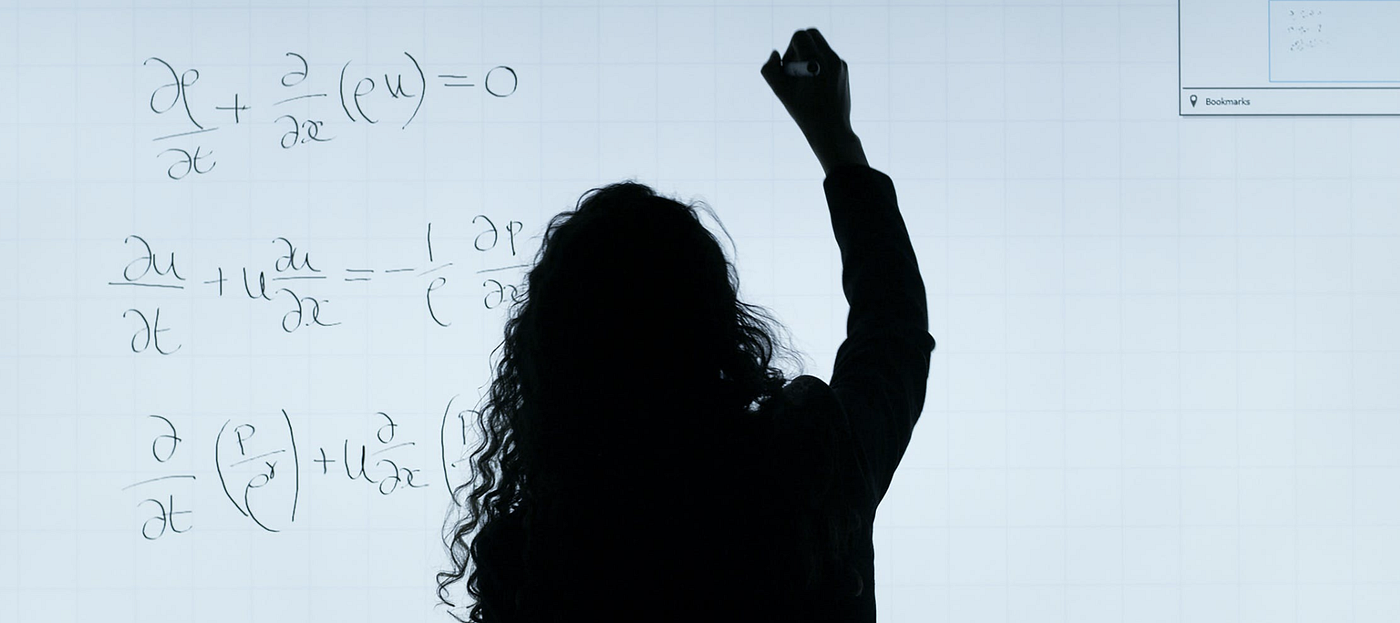1677936072

## Spearmint

Spearmint is a software package to perform Bayesian optimization. The Software is designed to automatically run experiments (thus the code name spearmint) in a manner that iteratively adjusts a number of parameters so as to minimize some objective in as few runs as possible.

### Relevant Publications

Spearmint implements a combination of the algorithms detailed in the following publications:

``````Practical Bayesian Optimization of Machine Learning Algorithms
Jasper Snoek, Hugo Larochelle and Ryan Prescott Adams
Advances in Neural Information Processing Systems, 2012

Kevin Swersky, Jasper Snoek and Ryan Prescott Adams
Advances in Neural Information Processing Systems, 2013

Input Warping for Bayesian Optimization of Non-stationary Functions
Jasper Snoek, Kevin Swersky, Richard Zemel and Ryan Prescott Adams
International Conference on Machine Learning, 2014

Bayesian Optimization and Semiparametric Models with Applications to Assistive Technology
Jasper Snoek, PhD Thesis, University of Toronto, 2013

Bayesian Optimization with Unknown Constraints
Michael Gelbart, Jasper Snoek and Ryan Prescott Adams
Uncertainty in Artificial Intelligence, 2014
``````

### Setting up Spearmint

STEP 1: Installation

1. Install python, numpy, scipy, pymongo. For academic users, the anaconda distribution is great. Use numpy 1.8 or higher. We use python 2.7.
3. Install the spearmint package using pip: `pip install -e \</path/to/spearmint/root\>` (the -e means changes will be reflected automatically)
5. Install the pymongo package using e.g., pip `pip install pymongo` or anaconda `conda install pymongo`

STEP 2: Setting up your experiment

1. Create a callable objective function. See `./examples/simple/branin.py` as an example
2. Create a config file. There are 3 example config files in the ../examples directory. Note 1: There are more parameters that can be set in the config files than what is shown in the examples, but these parameters all have default values. Note 2: By default Spearmint assumes your function is noisy (non-deterministic). If it is noise-free, you should set this explicitly as in the ../examples/simple/config.json file.

STEP 3: Running spearmint

1. Start up a MongoDB daemon instance:
`mongod --fork --logpath <path/to/logfile\> --dbpath <path/to/dbfolder\>`
2. Run spearmint: `python main.py \</path/to/experiment/directory\>`

STEP 4: Looking at your results
Spearmint will output results to standard out / standard err. You can also load the results from the database and manipulate them directly.

IMPORTANT: Spearmint is under an Academic and Non-Commercial Research Use License. Before using spearmint please be aware of the license. If you do not qualify to use spearmint you can ask to obtain a license as detailed in the license or you can use the older open source code version (which is somewhat outdated) at https://github.com/JasperSnoek/spearmint.

Author: HIPS
Source Code: https://github.com/HIPS/Spearmint1676766120

## Causalnex

A Python library that helps data scientists to infer causation rather than observing correlation.

## What is CausalNex?

"A toolkit for causal reasoning with Bayesian Networks."

CausalNex aims to become one of the leading libraries for causal reasoning and "what-if" analysis using Bayesian Networks. It helps to simplify the steps:

• To learn causal structures,
• To allow domain experts to augment the relationships,
• To estimate the effects of potential interventions using data.

## Why CausalNex?

CausalNex is built on our collective experience to leverage Bayesian Networks to identify causal relationships in data so that we can develop the right interventions from analytics. We developed CausalNex because:

• We believe leveraging Bayesian Networks is more intuitive to describe causality compared to traditional machine learning methodology that are built on pattern recognition and correlation analysis.
• Causal relationships are more accurate if we can easily encode or augment domain expertise in the graph model.
• We can then use the graph model to assess the impact from changes to underlying features, i.e. counterfactual analysis, and identify the right intervention.

In our experience, a data scientist generally has to use at least 3-4 different open-source libraries before arriving at the final step of finding the right intervention. CausalNex aims to simplify this end-to-end process for causality and counterfactual analysis.

## What are the main features of CausalNex?

The main features of this library are:

• Use state-of-the-art structure learning methods to understand conditional dependencies between variables
• Allow domain knowledge to augment model relationship
• Build predictive models based on structural relationships
• Fit probability distribution of the Bayesian Networks
• Evaluate model quality with standard statistical checks
• Simplify how causality is understood in Bayesian Networks through visualisation
• Analyse the impact of interventions using Do-calculus

## How do I install CausalNex?

CausalNex is a Python package. To install it, simply run:

``````pip install causalnex
``````

Since pygraphviz can be difficult to install, esp. on Windows machines, the requirement is optional. If you want to use the causalnex native plotting tools, you can use

``````pip install "causalnex[plot]"
``````

Alternatively, you can use the `networkx` drawing functionality for visualisations with fewer dependencies.

Use `all` for a full installation of dependencies (only the plotting right now):

``````pip install "causalnex[all]"
``````

See more detailed installation instructions, including how to setup Python virtual environments, in our installation guide and get started with our tutorial.

## How do I use CausalNex?

You can find the documentation for the latest stable release here. It explains:

Note: You can find the notebook and markdown files used to build the docs in `docs/source`.

## Can I contribute?

Yes! We'd love you to join us and help us build CausalNex. Check out our contributing documentation.

## How do I upgrade CausalNex?

We use SemVer for versioning. The best way to upgrade safely is to check our release notes for any notable breaking changes.

## How do I cite CausalNex?

You may click "Cite this repository" under the "About" section of this repository to get the citation information in APA and BibTeX formats.

## We're hiring!

Do you want to be part of the team that builds CausalNex and other great products at QuantumBlack? If so, you're in luck! QuantumBlack is currently hiring Machine Learning Engineers who love using data to drive their decisions. Take a look at our open positions and see if you're a fit.

Author: Quantumblacklabs
Source Code: https://github.com/quantumblacklabs/causalnex1671791422

## Metropolis-Hastings and Bayesian Inference

Markov Chain Monte Carlo (MCMC) methods let us compute samples from a distribution even though we can’t do this relying on traditional methods. In this article, Toptal Data Scientist Divyanshu Kalra will introduce you to Bayesian methods and Metropolis-Hastings, demonstrating their potential in the field of probabilistic programming.

Let’s get the basic definition out of the way: Markov Chain Monte Carlo (MCMC) methods let us compute samples from a distribution even though we can’t compute it.

What does this mean? Let’s back up and talk about Monte Carlo Sampling.

## Monte Carlo Sampling

What are Monte Carlo methods?

“Monte Carlo methods, or Monte Carlo experiments, are a broad class of computational algorithms that rely on repeated random sampling to obtain numerical results.” (Wikipedia)

Let’s break that down.

### Example 1: Area Estimation

Imagine that you have an irregular shape, like the shape presented below:And you are tasked with determining the area enclosed by this shape. One of the methods you can use is to make small squares in the shape, count the squares, and that will give you a pretty accurate approximation of the area. However, that is difficult and time-consuming.

Monte Carlo sampling to the rescue!

First, we draw a big square of a known area around the shape, for example of 50 cm2. Now we “hang” this square and start throwing darts randomly at the shape.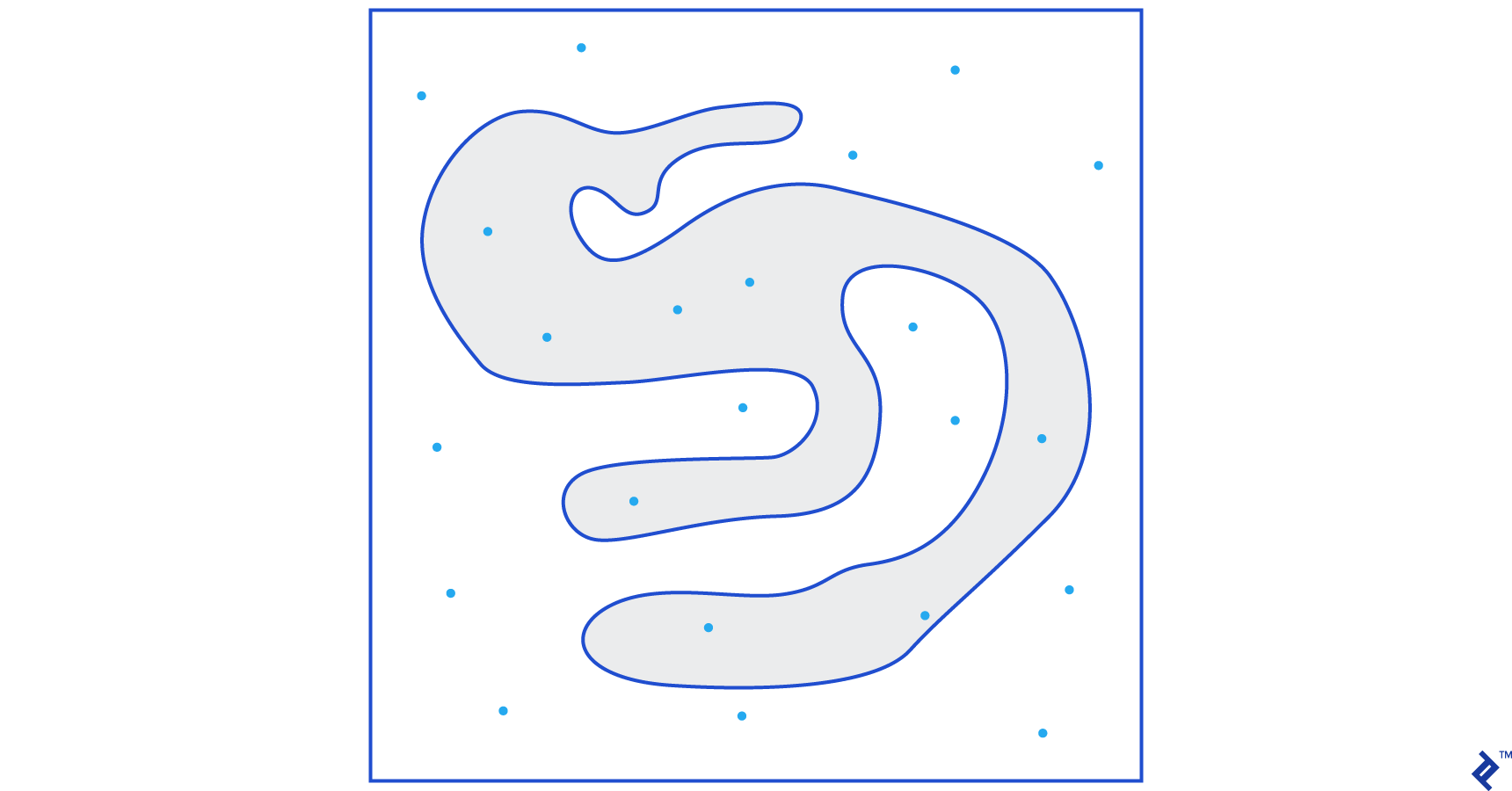Next, we count the total number of darts in the rectangle and the number of darts in the shape we are interested in. Let’s assume that the total number of “darts” used was 100 and that 22 of them ended up within the shape. Now the area can be calculated by the simple formula:

area of shape = area of square *(number of darts in the shape) / (number of darts in the square)

So, in our case, this comes down to:

area of shape = 50 * 22/100 = 11 cm2

If we multiply the number of “darts” by a factor of 10, this approximation becomes very close to the real answer: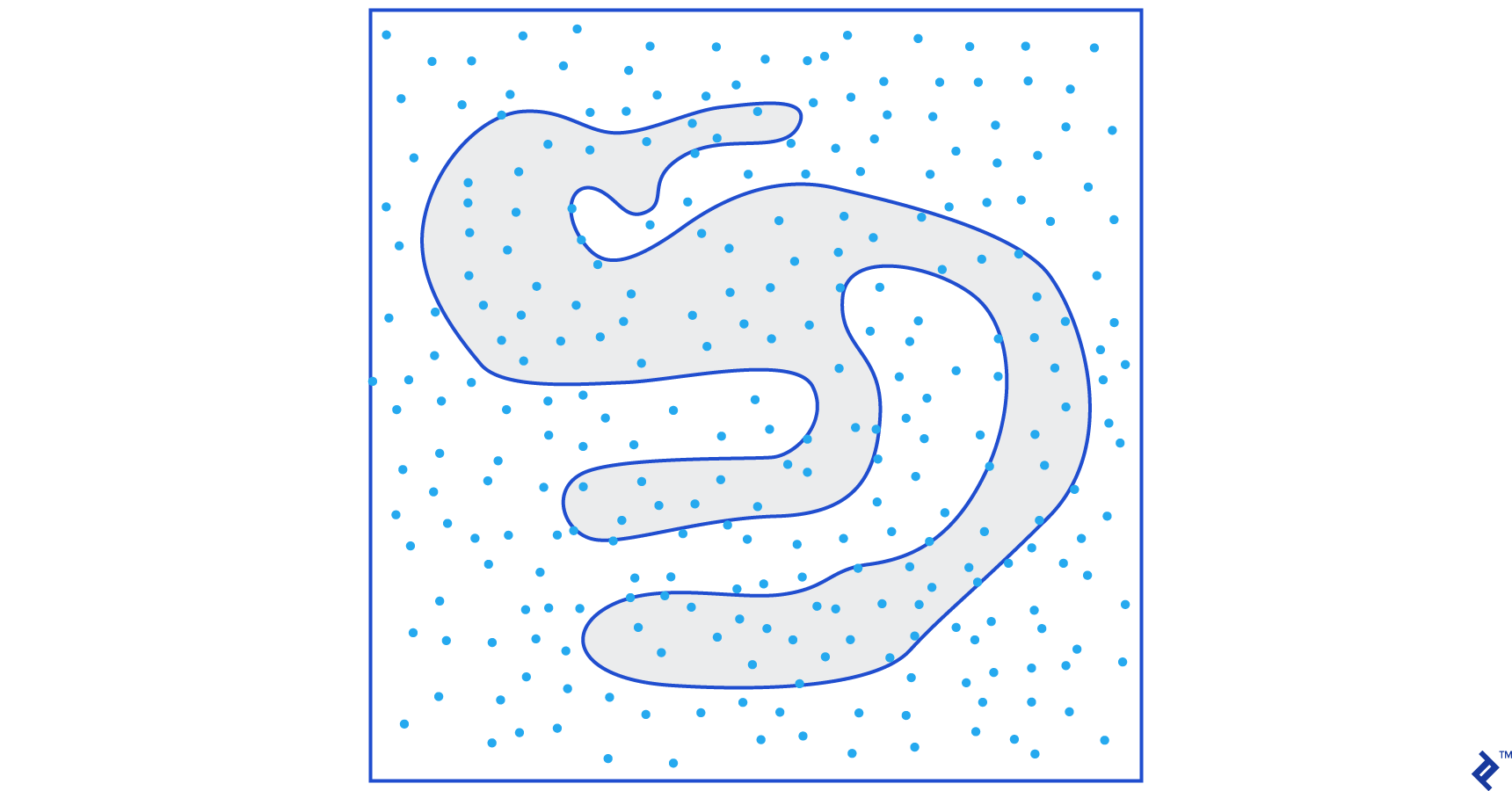area of shape = 50 * 280/1000 = 14 cm2

This is how we break down complicated tasks, like the one given above, by using Monte Carlo sampling.

### The Law of Large Numbers

The area approximation was closer the more darts we threw, and this is because of the Law of Large Numbers:

“The law of large numbers is a theorem that describes the result of performing the same experiment a large number of times. According to the law, the average of the results obtained from a large number of trials should be close to the expected value, and will tend to become closer as more trials are performed.”

This brings us to our next example, the famous Monty Hall problem.

### Example 2: The Monty Hall Problem

The Monty Hall problem is a very famous brain teaser:“There are three doors, one has a car behind it, the others have a goat behind them. You choose a door, the host opens a different door, and shows you there’s a goat behind it. He then asks you if you want to change your decision. Do you? Why? Why not?”

The first thing that comes to your mind is that the chances of winning are equal whether you switch or don’t, but that’s not true. Let’s make a simple flowchart to demonstrate the same.

Assuming that the car is behind door 3: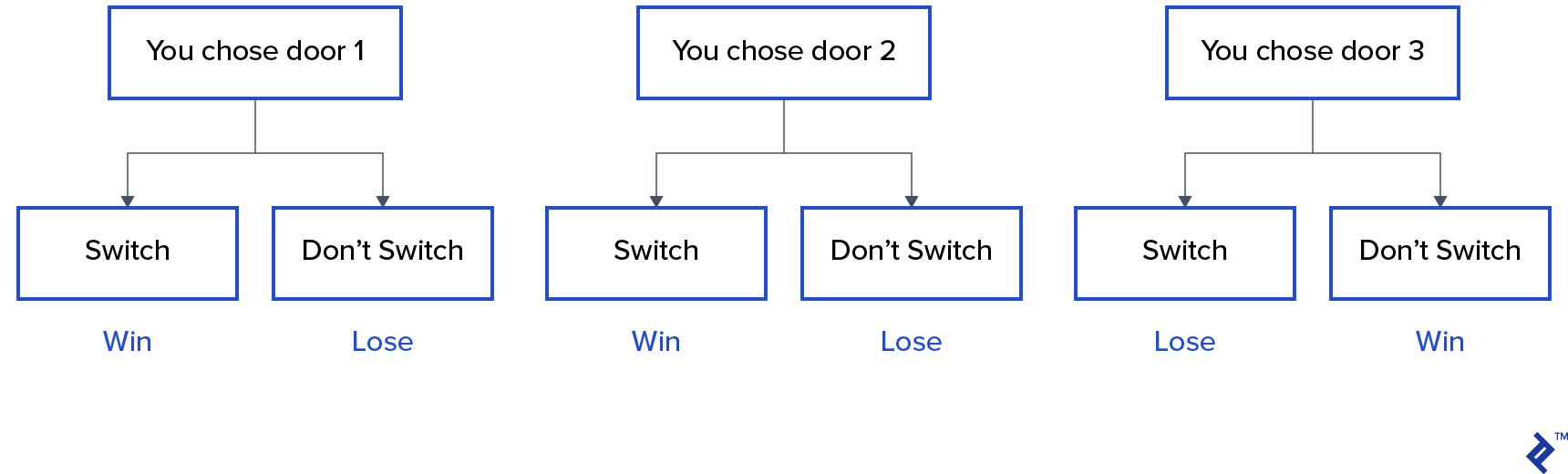Hence, if you switch, you win ⅔ times, and if you don’t switch, you win only ⅓ times.

Let’s solve this by using sampling.

``````
wins = []

for i in range(int(10e6)):

car_door = assign_car_door()

choice = random.randint(0, 2)

opened_door = assign_door_to_open(car_door)

did_he_win = win_or_lose(choice, car_door, opened_door, switch = False)

wins.append(did_he_win)

print(sum(wins)/len(wins))

``````

The `assign_car_door()` function is just a random number generator that selects a door 0, 1, or 2, behind which there is a car. Using `assign_door_to_open` selects a door that has a goat behind it and is not the one you selected, and the host opens it. `win_or_lose` returns true or false, denoting whether you won the car or not, it takes a bool “switch” which says whether you switched the door or not.

Let’s run this simulation 10 million times:

• Probability of winning if you don’t switch: 0.334134
• Probability of winning if you do switch: 0.667255

This is very close to the answers the flowchart gave us.

In fact, the more we run this simulation, the closer the answer comes to the true value, hence validating the law of large numbers: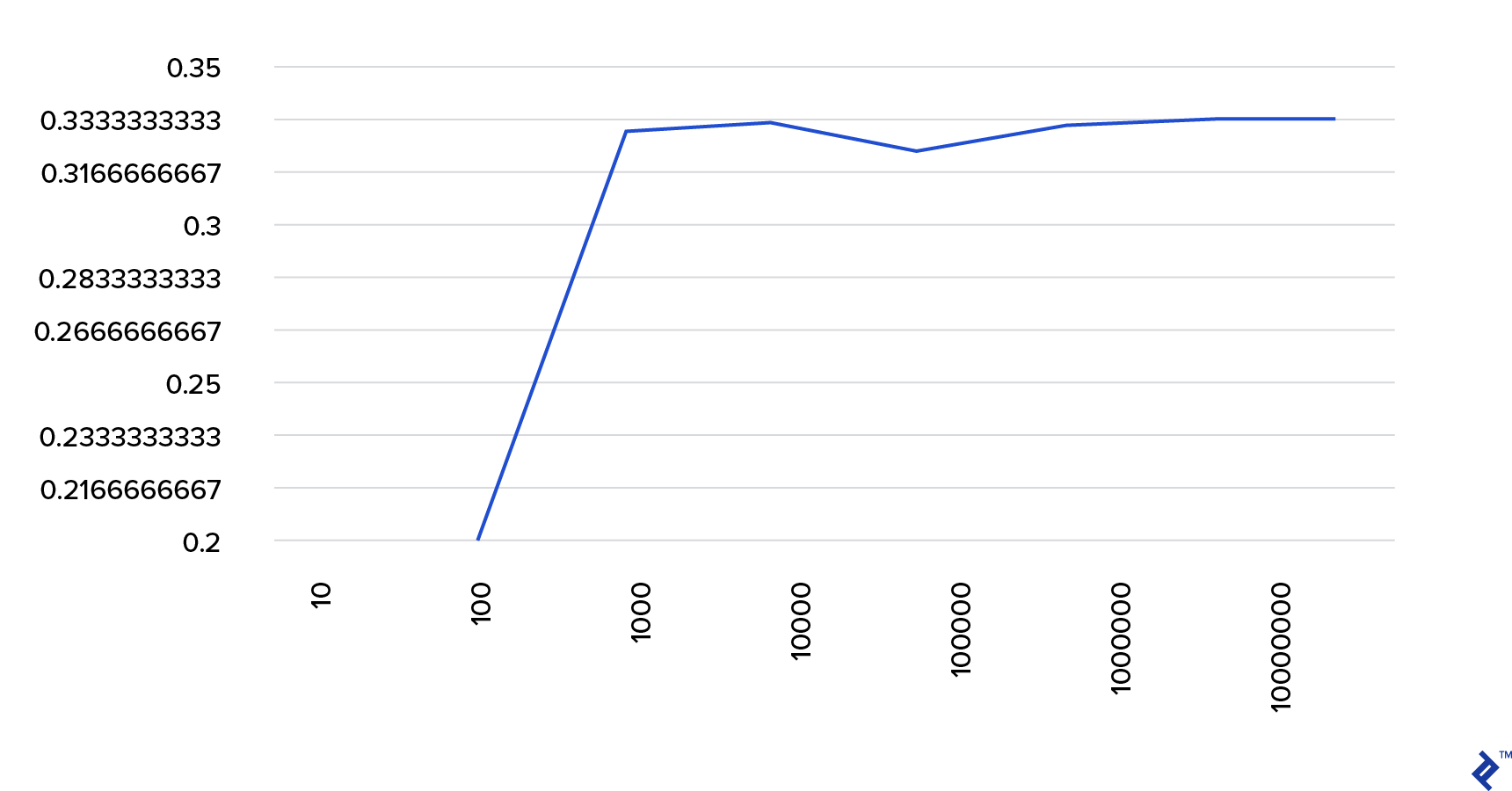The x-axis is the number of simulations run, and y is the probability of winning if you don’t switch.

The same can be seen from this table:

## The Bayesian Way of Thinking

“Frequentist, known as the more classical version of statistics, assume that probability is the long-run frequency of events (hence the bestowed title).”

“Bayesian statistics is a theory in the field of statistics based on the Bayesian interpretation of probability where probability expresses a degree of belief in an event. The degree of belief may be based on prior knowledge about the event, such as the results of previous experiments, or on personal beliefs about the event.” - from Probabilistic Programming and Bayesian Methods for Hackers

What does this mean?

In the frequentist way of thinking, we look at probabilities in the long run. When a frequentist says that there is a 0.001% chance of a car crash happening, it means, if we consider infinite car trips, 0.001% of them will end in a crash.

A Bayesian mindset is different, as we start with a prior, a belief. If we talk about a belief of 0, it means that your belief is that the event will never happen; conversely, a belief of 1 means that you are sure it will happen.

Then, once we start observing data, we update this belief to take into consideration the data. How do we do this? By using the Bayes theorem.

### Bayes Theorem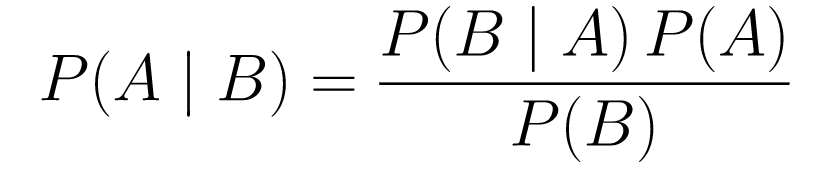....(1)

Let’s break it down.

• `P(A | B)` gives us the probability of event A given event B. This is the posterior, B is the data we observed, so we are essentially saying what the probability of an event happening is, considering the data we observed.
• `P(A)` is the prior, our belief that event A will happen.
• `P(B | A)` is the likelihood, what is the probability that we will observe the data given that A is true.

Let’s look at an example, the cancer screening test.

#### PROBABILITY OF CANCER

Let’s say a patient goes to get a mammogram done, and the mammogram comes back positive. What is the probability that the patient actually has cancer?

Let’s define the probabilities:

• 1% of women have breast cancer.
• Mammograms detect cancer 80% of the time when it is actually present.
• 9.6% of mammograms falsely report that you have cancer when you actually do not have it.

So, if you were to say that if a mammogram came back positive meaning there is an 80% chance that you have cancer, that would be wrong. You would not take into consideration that having cancer is a rare event, i.e., that only 1% of women have breast cancer. We need to take this as prior, and this is where the Bayes theorem comes into play:

P(C+ | T+) =(P(T+|C+)*P(C+))/P(T+)

• `P(C+ | T+)`: This is the probability that cancer is there, given that the test was positive, this is what we are interested in.
• `P(T+ | C+)`: This is the probability that the test is positive, given that there is cancer, this, as discussed above is equal to 80% = 0.8.
• `P(C+)`: This is the prior probability, the probability of an individual having cancer, which is equal to 1% = 0.01.
• `P(T+)`: This is the probability that the test is positive, no matter what, so it has two components: P(T+) = P(T+|C-)P(C-)+P(T+|C+)P(C+)
• `P(T+ | C-)`: This is the probability that the test came back positive but there is no cancer, this is given by 0.096.
• `P(C-)`: This is the probability of not having cancer since the probability of having cancer is 1%, this is equal to 99% = 0.99.
• `P(T+ | C+)`: This is the probability that the test came back positive, given that you have cancer, this is equal to 80% = 0.8.
• `P(C+)`: This is the probability of having cancer, which is equal to 1% = 0.01.

Plugging all of this into the original formula: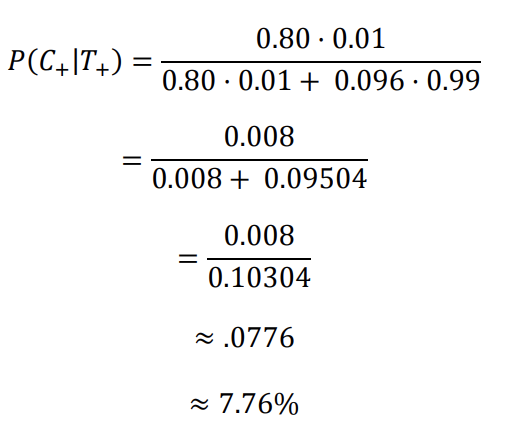So, given that the mammogram came back positive, there is a 7.76% chance of the patient having cancer. It might seem strange at first but it makes sense. The test gives a false positive 9.6% of the time (quite high), so there will be many false positives in a given population. For a rare disease, most of the positive test results will be wrong.

Let us now revisit the Monty Hall problem and solve it using the Bayes theorem.

#### MONTY HALL PROBLEM

The priors can be defined as:

• Assume you choose door A as your choice in the beginning.
• P(H) = ⅓, ⅓. ⅓ for all three doors, which means that before the door was open and the goat revealed, there was an equal chance of the car being behind any of them.

The likelihood can be defined as:

• `P(D|H)`, where event D is that Monty chooses door B and there is no car behind it.
• `P(D|H)` = ½ if the car is behind door A - since there is a 50% chance that he will choose door B and 50% chance that he chooses door C.
• `P(D|H)` = 0 if the car is behind door B, since there is a 0% chance that he will choose door B if the car is behind it.
• `P(D|H)` = 1 if the car is behind C, and you choose A, there is a 100% probability that he will choose door B.

We are interested in `P(H|D)`, which is the probability that the car is behind a door given that it shows us a goat behind one of the other doors.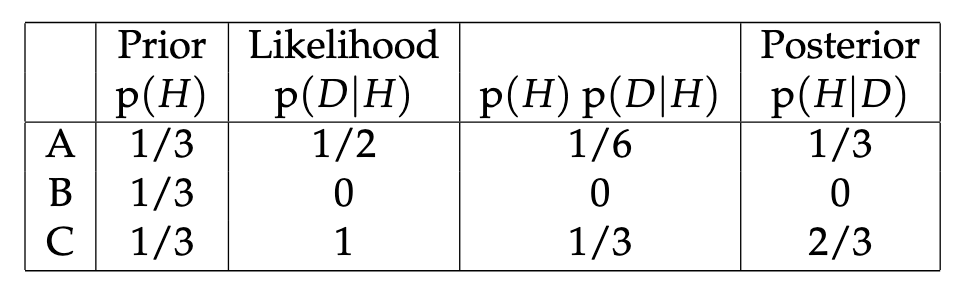It can be seen from the posterior, `P(H|D)`, that switching to door C from A will increase the probability of winning.

## Metropolis-Hastings

Now, let’s combine everything we covered so far and try to understand how Metropolis-Hastings works.

Metropolis-Hastings uses the Bayes theorem to get the posterior distribution of a complex distribution, from which sampling directly is difficult.

How? Essentially, it randomly selects different samples from a space and checks if the new sample is more probable of being from the posterior than the last sample, since we are looking at the ratio of probabilities, P(B) in equation (1), gets canceled out:

P(acceptance) = ( P(newSample) * Likelihood of new sample) / (P(oldSample) * Likelihood of old sample)

The “likelihood” of each new sample is decided by the function f. That’s why f must be proportional to the posterior we want to sample from.

To decide if θ′ is to be accepted or rejected, the following ratio must be computed for each new proposed θ’: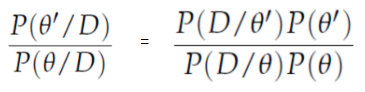Where θ is the old sample, `P(D| θ)` is the likelihood of sample θ.

Let’s use an example to understand this better. Let’s say you have data but you want to find out the normal distribution that fits it, so:

X ~ N(mean, std)

Now we need to define the priors for the mean and std both. For simplicity, we will assume that both follow a normal distribution with mean 1 and std 2:

Mean ~ N(1, 2)
std ~ N(1, 2)

Now, let’s generate some data and assume the true mean and std are 0.5 and 1.2, respectively.

``````
import numpy as np

import matplotlib.pyplot as plt

import scipy.stats as st

meanX = 1.5

stdX = 1.2

X = np.random.normal(meanX, stdX, size = 1000)

_ = plt.hist(X, bins = 50)

``````

### PyMC3

Let’s first use a library called PyMC3 to model the data and find the posterior distribution for the mean and std.

``````
import pymc3 as pm

with pm.Model() as model:

mean = pm.Normal("mean", mu = 1, sigma = 2)

std = pm.Normal("std", mu = 1, sigma = 2)

x = pm.Normal("X", mu = mean, sigma = std, observed = X)

step = pm.Metropolis()

trace = pm.sample(5000, step = step)

``````

Let’s go over the code.

First, we define the prior for mean and std, i.e., a normal with mean 1 and std 2.

x = pm.Normal(“X”, mu = mean, sigma = std, observed = X)

In this line, we define the variable that we are interested in; it takes the mean and std we defined earlier, it also takes observed values that we calculated earlier.

Let’s look at the results:

``````
_ = plt.hist(trace['std'], bins = 50, label = "std")

_ = plt.hist(trace['mean'], bins = 50, label = "mean")

_ = plt.legend()

``````

The std is centered around 1.2, and the mean is at 1.55 - pretty close!

Let’s now do it from scratch to understand Metropolis-Hastings.

## From Scratch

### Generating a Proposal

First, let’s understand what Metropolis-Hastings does. Earlier in this article, we said, “Metropolis-Hastings randomly selects different samples from a space,” but how does it know which sample to select?

It does so using the proposal distribution. It is a normal distribution centered at the currently accepted sample and has an STD of 0.5. Where 0.5 is a hyperparameter, we can tweak; the more the STD of the proposal, the further it will search from the currently accepted sample. Let’s code this.

Since we have to find the mean and std of the distribution, this function will take the currently accepted mean and the currently accepted std, and return proposals for both.

``````
def get_proposal(mean_current, std_current, proposal_width = 0.5):

return np.random.normal(mean_current, proposal_width), \

np.random.normal(std_current, proposal_width)

``````

### Accepting/Rejecting a Proposal

Now, let’s code the logic that accepts or rejects the proposal. It has two parts: the prior and the likelihood.

First, let’s calculate the probability of the proposal coming from the prior:

``````
def prior(mean, std, prior_mean, prior_std):

return st.norm(prior_mean, prior_mean).pdf(mean)* \

st.norm(prior_std, prior_std).pdf(std)

``````

It just takes the mean and std and calculates how likely it is that this mean and std came from the prior distribution that we defined.

In calculating the likelihood, we calculate how likely it is that the data that we saw came from the proposed distribution.

``````
def likelihood(mean, std, data):

return np.prod(st.norm(mean, std).pdf(X))

``````

So for each data point, we multiply the probability of that data point coming from the proposed distribution.

Now, we need to call these functions for the current mean and std and for the proposed mean and std.

``````
prior_current = prior(mean_current, std_current, prior_mean, prior_std)

likelihood_current = likelihood(mean_current, std_current, data)

prior_proposal = prior(mean_proposal, std_proposal, prior_mean, prior_std)

likelihood_proposal = likelihood(mean_proposal, std_proposal, data)

``````

The whole code:

``````
def accept_proposal(mean_proposal, std_proposal, mean_current, \

std_current, prior_mean, prior_std, data):

def prior(mean, std, prior_mean, prior_std):

return st.norm(prior_mean, prior_mean).pdf(mean)* \

st.norm(prior_std, prior_std).pdf(std)

def likelihood(mean, std, data):

return np.prod(st.norm(mean, std).pdf(X))

prior_current = prior(mean_current, std_current, prior_mean, prior_std)

likelihood_current = likelihood(mean_current, std_current, data)

prior_proposal = prior(mean_proposal, std_proposal, prior_mean, prior_std)

likelihood_proposal = likelihood(mean_proposal, std_proposal, data)

return (prior_proposal * likelihood_proposal) / (prior_current * likelihood_current)

``````

Now, let’s create the final function that will tie everything together and generate the posterior samples we need.

### Generating the Posterior

Now, we call the functions we have written above and generate the posterior!

``````
def get_trace(data, samples = 5000):

mean_prior = 1

std_prior = 2

mean_current = mean_prior

std_current = std_prior

trace = {

"mean": [mean_current],

"std": [std_current]

}

for i in tqdm(range(samples)):

mean_proposal, std_proposal = get_proposal(mean_current, std_current)

acceptance_prob = accept_proposal(mean_proposal, std_proposal, mean_current, \

std_current, [mean_prior, std_prior], \

[mean_prior, std_prior], data)

if np.random.rand() < acceptance_prob:

mean_current = mean_proposal

std_current = std_proposal

trace['mean'].append(mean_current)

trace['std'].append(std_current)

return trace

``````

### Room for Improvement

Recall `a * b = antilog(log(a) + log(b))` and `a / b = antilog(log(a) - log(b)).`

This is beneficial to us because we will be dealing with very small probabilities, multiplying which will result in zero, so we will rather add the log prob, problem solved!

So, our previous code becomes:

``````
def accept_proposal(mean_proposal, std_proposal, mean_current, \

std_current, prior_mean, prior_std, data):

def prior(mean, std, prior_mean, prior_std):

return st.norm(prior_mean, prior_mean).logpdf(mean)+ \

st.norm(prior_std, prior_std).logpdf(std)

def likelihood(mean, std, data):

return np.sum(st.norm(mean, std).logpdf(X))

prior_current = prior(mean_current, std_current, prior_mean, prior_std)

likelihood_current = likelihood(mean_current, std_current, data)

prior_proposal = prior(mean_proposal, std_proposal, prior_mean, prior_std)

likelihood_proposal = likelihood(mean_proposal, std_proposal, data)

return (prior_proposal + likelihood_proposal) - (prior_current + likelihood_current)

``````

Since we are returning the log of probability of acceptance:

`if np.random.rand() < acceptance_prob:`

Becomes:

`if math.log(np.random.rand()) < acceptance_prob:`

Let’s run the new code and plot the results.

``````
_ = plt.hist(trace['std'], bins = 50, label = "std")

_ = plt.hist(trace['mean'], bins = 50, label = "mean")

_ = plt.legend()

``````

As you can see, the std is centered at 1.2, and the mean at 1.5. We did it!

## Moving Forward

By now, you hopefully understand how Metropolis-Hastings works and you might be wondering where you can use it.

Well, Bayesian Methods for Hackers is an excellent book that explains probabilistic programming, and if you want to learn more about the Bayes theorem and its applications, Think Bayes is a great book by Allen B. Downey.

Thank you for reading, and I hope this article encourages you to discover the amazing world of Bayesian stats.

Original article source at: https://www.toptal.com/1667492100

## BayestestR

Become a Bayesian master you will

⚠️ We changed the default the CI width! Please make an informed decision and set it explicitly (`ci = 0.89`, `ci = 0.95` or anything else that you decide) ⚠️

Existing R packages allow users to easily fit a large variety of models and extract and visualize the posterior draws. However, most of these packages only return a limited set of indices (e.g., point-estimates and CIs). bayestestR provides a comprehensive and consistent set of functions to analyze and describe posterior distributions generated by a variety of models objects, including popular modeling packages such as rstanarm, brms or BayesFactor.

You can reference the package and its documentation as follows:

• Makowski, D., Ben-Shachar, M. S., & Lüdecke, D. (2019). bayestestR: Describing Effects and their Uncertainty, Existence and Significance within the Bayesian Framework. Journal of Open Source Software, 4(40), 1541. 10.21105/joss.01541
• Makowski, D., Ben-Shachar, M. S., Chen, S. H. A., & Lüdecke, D. (2019). Indices of Effect Existence and Significance in the Bayesian Framework. Frontiers in Psychology 2019;10:2767. 10.3389/fpsyg.2019.02767

## Installation

The bayestestR package is available on CRAN, while its latest development version is available on R-universe (from rOpenSci).

``````library("bayestestR")
``````

Tip

Instead of `library(datawizard)`, use `library(easystats)`. This will make all features of the easystats-ecosystem available.

To stay updated, use `easystats::install_latest()`.

## Documentation

Access the package documentation and check-out these vignettes:

### Articles

Features

In the Bayesian framework, parameters are estimated in a probabilistic fashion as distributions. These distributions can be summarised and described by reporting four types of indices:

`describe_posterior()` is the master function with which you can compute all of the indices cited below at once.

``````describe_posterior(
rnorm(10000),
centrality = "median",
test = c("p_direction", "p_significance")
)
## Summary of Posterior Distribution
##
## Parameter |    Median |        95% CI |     pd |   ps
## -----------------------------------------------------
## Posterior | -4.19e-03 | [-1.91, 1.98] | 50.18% | 0.46
``````

`describe_posterior()` works for many objects, including more complex brmsfit-models. For better readability, the output is separated by model components:

``````zinb <- read.csv("http://stats.idre.ucla.edu/stat/data/fish.csv")
set.seed(123)
model <- brm(
bf(
count ~ child + camper + (1 | persons),
zi ~ child + camper + (1 | persons)
),
data = zinb,
family = zero_inflated_poisson(),
chains = 1,
iter = 500
)

describe_posterior(
model,
effects = "all",
component = "all",
test = c("p_direction", "p_significance"),
centrality = "all"
)
``````
``````## Summary of Posterior Distribution
##
## Parameter   | Median |  Mean |   MAP |         95% CI |     pd |   ps |  Rhat |    ESS
## --------------------------------------------------------------------------------------
## (Intercept) |   0.96 |  0.96 |  0.96 | [-0.81,  2.51] | 90.00% | 0.88 | 1.011 | 110.00
## child       |  -1.16 | -1.16 | -1.16 | [-1.36, -0.94] |   100% | 1.00 | 0.996 | 278.00
## camper      |   0.73 |  0.72 |  0.73 | [ 0.54,  0.91] |   100% | 1.00 | 0.996 | 271.00
##
## # Fixed effects (zero-inflated)
##
## Parameter   | Median |  Mean |   MAP |         95% CI |     pd |   ps |  Rhat |    ESS
## --------------------------------------------------------------------------------------
## (Intercept) |  -0.48 | -0.51 | -0.22 | [-2.03,  0.89] | 78.00% | 0.73 | 0.997 | 138.00
## child       |   1.85 |  1.86 |  1.81 | [ 1.19,  2.54] |   100% | 1.00 | 0.996 | 303.00
## camper      |  -0.88 | -0.86 | -0.99 | [-1.61, -0.07] | 98.40% | 0.96 | 0.996 | 292.00
##
## # Random effects (conditional) Intercept: persons
##
## Parameter |    Median |  Mean |   MAP |         95% CI |     pd |   ps |  Rhat |    ESS
## ---------------------------------------------------------------------------------------
## persons.1 |     -0.99 | -1.01 | -0.84 | [-2.68,  0.80] | 92.00% | 0.90 | 1.007 | 106.00
## persons.2 | -4.65e-03 | -0.04 |  0.03 | [-1.63,  1.66] | 50.00% | 0.45 | 1.013 | 109.00
## persons.3 |      0.69 |  0.66 |  0.69 | [-0.95,  2.34] | 79.60% | 0.78 | 1.010 | 114.00
## persons.4 |      1.57 |  1.56 |  1.56 | [-0.05,  3.29] | 96.80% | 0.96 | 1.009 | 114.00
##
## # Random effects (zero-inflated) Intercept: persons
##
## Parameter | Median |  Mean |   MAP |         95% CI |     pd |   ps |  Rhat |    ESS
## ------------------------------------------------------------------------------------
## persons.1 |   1.10 |  1.11 |  1.08 | [-0.23,  2.72] | 94.80% | 0.93 | 0.997 | 166.00
## persons.2 |   0.18 |  0.18 |  0.22 | [-0.94,  1.58] | 63.20% | 0.54 | 0.996 | 154.00
## persons.3 |  -0.30 | -0.31 | -0.54 | [-1.79,  1.02] | 64.00% | 0.59 | 0.997 | 154.00
## persons.4 |  -1.45 | -1.46 | -1.44 | [-2.90, -0.10] | 98.00% | 0.97 | 1.000 | 189.00
##
## # Random effects (conditional) SD/Cor: persons
##
## Parameter   | Median | Mean |  MAP |         95% CI |   pd |   ps |  Rhat |    ESS
## ----------------------------------------------------------------------------------
## (Intercept) |   1.42 | 1.58 | 1.07 | [ 0.71,  3.58] | 100% | 1.00 | 1.010 | 126.00
##
## # Random effects (zero-inflated) SD/Cor: persons
##
## Parameter   | Median | Mean |  MAP |         95% CI |   pd |   ps |  Rhat |    ESS
## ----------------------------------------------------------------------------------
## (Intercept) |   1.30 | 1.49 | 0.99 | [ 0.63,  3.41] | 100% | 1.00 | 0.996 | 129.00
``````

bayestestR also includes many other features useful for your Bayesian analsyes. Here are some more examples:

## Point-estimates

``````library(bayestestR)

posterior <- distribution_gamma(10000, 1.5) # Generate a skewed distribution
centrality <- point_estimate(posterior) # Get indices of centrality
centrality
## Point Estimate
##
## Median | Mean |  MAP
## --------------------
## 1.18   | 1.50 | 0.51
``````

As for other easystats packages, `plot()` methods are available from the see package for many functions:

While the median and the mean are available through base R functions, `map_estimate()` in bayestestR can be used to directly find the Highest Maximum A Posteriori (MAP) estimate of a posterior, i.e., the value associated with the highest probability density (the “peak” of the posterior distribution). In other words, it is an estimation of the mode for continuous parameters.

## Uncertainty (CI)

`hdi()` computes the Highest Density Interval (HDI) of a posterior distribution, i.e., the interval which contains all points within the interval have a higher probability density than points outside the interval. The HDI can be used in the context of Bayesian posterior characterization as Credible Interval (CI).

Unlike equal-tailed intervals (see `eti()`) that typically exclude 2.5% from each tail of the distribution, the HDI is not equal-tailed and therefore always includes the mode(s) of posterior distributions.

``````posterior <- distribution_chisquared(10000, 4)

hdi(posterior, ci = 0.89)
## 89% HDI: [0.18, 7.63]

eti(posterior, ci = 0.89)
## 89% ETI: [0.75, 9.25]
``````

## Existence and Significance Testing

### Probability of Direction (pd)

`p_direction()` computes the Probability of Direction (pd, also known as the Maximum Probability of Effect - MPE). It varies between 50% and 100% (i.e., `0.5` and `1`) and can be interpreted as the probability (expressed in percentage) that a parameter (described by its posterior distribution) is strictly positive or negative (whichever is the most probable). It is mathematically defined as the proportion of the posterior distribution that is of the median’s sign. Although differently expressed, this index is fairly similar (i.e., is strongly correlated) to the frequentist p-value.

Relationship with the p-value: In most cases, it seems that the pd corresponds to the frequentist one-sided p-value through the formula `p-value = (1-pd/100)` and to the two-sided p-value (the most commonly reported) through the formula `p-value = 2*(1-pd/100)`. Thus, a `pd` of `95%`, `97.5%` `99.5%` and `99.95%` corresponds approximately to a two-sided p-value of respectively `.1`, `.05`, `.01` and `.001`. See the reporting guidelines.

``````posterior <- distribution_normal(10000, 0.4, 0.2)
p_direction(posterior)
## Probability of Direction: 0.98
``````

### ROPE

`rope()` computes the proportion (in percentage) of the HDI (default to the 89% HDI) of a posterior distribution that lies within a region of practical equivalence.

Statistically, the probability of a posterior distribution of being different from 0 does not make much sense (the probability of it being different from a single point being infinite). Therefore, the idea underlining ROPE is to let the user define an area around the null value enclosing values that are equivalent to the null value for practical purposes Kruschke (2018).

Kruschke suggests that such null value could be set, by default, to the -0.1 to 0.1 range of a standardized parameter (negligible effect size according to Cohen, 1988). This could be generalized: For instance, for linear models, the ROPE could be set as `0 +/- .1 * sd(y)`. This ROPE range can be automatically computed for models using the rope_range function.

Kruschke suggests using the proportion of the 95% (or 90%, considered more stable) HDI that falls within the ROPE as an index for “null-hypothesis” testing (as understood under the Bayesian framework, see equivalence_test).

``````posterior <- distribution_normal(10000, 0.4, 0.2)
rope(posterior, range = c(-0.1, 0.1))
## # Proportion of samples inside the ROPE [-0.10, 0.10]:
##
## inside ROPE
## -----------
## 4.40 %
``````

### Bayes Factor

`bayesfactor_parameters()` computes Bayes factors against the null (either a point or an interval), bases on prior and posterior samples of a single parameter. This Bayes factor indicates the degree by which the mass of the posterior distribution has shifted further away from or closer to the null value(s) (relative to the prior distribution), thus indicating if the null value has become less or more likely given the observed data.

When the null is an interval, the Bayes factor is computed by comparing the prior and posterior odds of the parameter falling within or outside the null; When the null is a point, a Savage-Dickey density ratio is computed, which is also an approximation of a Bayes factor comparing the marginal likelihoods of the model against a model in which the tested parameter has been restricted to the point null (Wagenmakers, Lodewyckx, Kuriyal, & Grasman, 2010).

``````prior <- distribution_normal(10000, mean = 0, sd = 1)
posterior <- distribution_normal(10000, mean = 1, sd = 0.7)

bayesfactor_parameters(posterior, prior, direction = "two-sided", null = 0)
## Bayes Factor (Savage-Dickey density ratio)
##
## BF
## ----
## 1.94
##
## * Evidence Against The Null: 0
``````

The lollipops represent the density of a point-null on the prior distribution (the blue lollipop on the dotted distribution) and on the posterior distribution (the red lollipop on the yellow distribution). The ratio between the two - the Savage-Dickey ratio - indicates the degree by which the mass of the parameter distribution has shifted away from or closer to the null.

## Utilities

### Find ROPE’s appropriate range

`rope_range()`: This function attempts at automatically finding suitable “default” values for the Region Of Practical Equivalence (ROPE). Kruschke (2018) suggests that such null value could be set, by default, to a range from `-0.1` to `0.1` of a standardized parameter (negligible effect size according to Cohen, 1988), which can be generalised for linear models to `-0.1 * sd(y), 0.1 * sd(y)`. For logistic models, the parameters expressed in log odds ratio can be converted to standardized difference through the formula `sqrt(3)/pi`, resulting in a range of `-0.05` to `0.05`.

``````rope_range(model)
``````

### Density Estimation

`estimate_density()`: This function is a wrapper over different methods of density estimation. By default, it uses the base R `density` with by default uses a different smoothing bandwidth (`"SJ"`) from the legacy default implemented the base R `density` function (`"nrd0"`). However, Deng & Wickham suggest that `method = "KernSmooth"` is the fastest and the most accurate.

### Perfect Distributions

`distribution()`: Generate a sample of size n with near-perfect distributions.

``````distribution(n = 10)
##   -1.55 -1.00 -0.66 -0.38 -0.12  0.12  0.38  0.66  1.00  1.55
``````

### Probability of a Value

`density_at()`: Compute the density of a given point of a distribution.

``````density_at(rnorm(1000, 1, 1), 1)
##  0.45
``````

## Code of Conduct

Please note that the bayestestR project is released with a Contributor Code of Conduct. By contributing to this project, you agree to abide by its terms.

References

Kruschke, J. K. (2018). Rejecting or accepting parameter values in Bayesian estimation. Advances in Methods and Practices in Psychological Science, 1(2), 270–280. https://doi.org/10.1177/2515245918771304

Kruschke, J. K., & Liddell, T. M. (2018). The bayesian new statistics: Hypothesis testing, estimation, meta-analysis, and power analysis from a bayesian perspective. Psychonomic Bulletin & Review, 25(1), 178–206.

Wagenmakers, E.-J., Lodewyckx, T., Kuriyal, H., & Grasman, R. (2010). Bayesian hypothesis testing for psychologists: A tutorial on the savage–dickey method. Cognitive Psychology, 60(3), 158–189.

Author: easystats
Source Code: https://github.com/easystats/bayestestR1667484240

## Bayesian Data Analysis R Demos

The BDA_R_demos repository contains some R demos and additional notes for the book Bayesian Data Analysis, 3rd ed by Gelman, Carlin, Stern, Dunson, Vehtari, and Rubin (BDA3). See also Bayesian Data Analysis course material.

Currently there are demos for BDA3 Chapters 2, 3, 4, 5, 6, 10, 11 and 12. Furthermore there are demos for CmdStanR, RStan RStanARM.

The initial demos were originally written for Matlab by Aki Vehtari and translated to R by Markus Paasiniemi. Recently more demos have been added for CmdStanR, RStan and RStanARM. Unless otherwise specified in specific files all code licensed under BSD-3 and all text, slides and figures licensed under CC-BY-NC 4.0.

The corresponding Python demos and the corresponding Matlab/Octave demos.

List of demos (not including rstan and rstanarm demos)

Author: Avehtari
Source Code: https://github.com/avehtari/BDA_R_demos1658327340

## #bayesian-bandit.js

This is an adaptation of the Bayesian Bandit code from Probabilistic Programming and Bayesian Methods for Hackers, specifically d3bandits.js.

The code has been rewritten to be more idiomatic and also usable as a browser script or npm package. Additionally, unit tests are included.

#Quick Start

From node.js:

``````npm install bayesian-bandit
node
``````

Then:

``````var Bandit = require('bayesian-bandit').Bandit
``````

In the browser:

``````<script src="https://raw.github.com/omphalos/bayesian-bandit.js/master/bayesian-bandit.js"></script>
``````

Sample JavaScript:

``````var bandit = new Bandit({ numberOfArms: 2 })

bandit.arms.reward(0) // Arm 0 loses once.
bandit.arms.reward(1) // Arm 0 wins once.

bandit.arms.reward(0) // Arm 1 loses once.
bandit.arms.reward(1) // Arm 1 wins once.
bandit.arms.reward(1) // Arm 1 wins twice.

bandit.selectArm() // Arm 1 is more likely, so this probably returns 1
``````

If you have pre-existing data that you want to load, you can explicitly pass in data via constructor. The following creates a bandit with 3 arms

``````var bandit = new Bandit({
arms: [{ count: 10, sum: 2 },
{ count: 20, sum: 10 },
{ count: 15: sum: 1}]
})
``````

You can also take advantage of the rewardMultiple function on the arms:

``````var bandit = new Bandit({ numberOfArms: 3 })

bandit.arms.rewardMultiple(10, 2)  // Arm 0 wins 2 of 10 times
bandit.arms.rewardMultiple(20, 10) // Arm 1 wins 10 of 20 times
bandit.arms.rewardMultiple(15, 1)  // Arm 2 wins 1 of 15 times
``````

#Unit Tests

The unit tests use nodeunit, which should get installed with:

``````npm install
``````

Then you can run unit tests with:

``npm test``

Author:  omphalos
Source code: https://github.com/omphalos/bayesian-bandit.js1622247780

## Bayesian Machine Learning - Exploring A Paradigm Shift In Statistical Data Modelling

### What is Bayesian Machine Learning?

Bayesian Machine Learning (also known as Bayesian ML) is a systematic approach to construct statistical models, based on Bayes’ Theorem.Any standard machine learning problem includes two primary datasets that need analysis:

1. A comprehensive set of training data
2. A collection of all available inputs and all recorded outputs

The traditional approach to analysing this data for modelling is to determine some patterns that can be mapped between these datasets. An analyst will usually splice together a model to determine the mapping between these, and the resultant approach is a very deterministic method to generate predictions for a target variable.

The only problem is that there is absolutely no way to explain what is happening inside this model with a clear set of definitions. All that is accomplished, essentially, is the minimisation of some loss functions on the training data set – but that hardly qualifies as true modelling.

An ideal (and preferably, lossless) model entails an objective summary of the model’s inherent parameters, supplemented with statistical easter eggs (such as confidence intervals) that can be defined and defended in the language of mathematical probability. This “ideal” scenario is what Bayesian Machine Learning sets out to accomplish.

### The Goals (And Magic) Of Bayesian Machine Learning

The primary objective of Bayesian Machine Learning is to estimate the posterior distribution, given the likelihood (a derivative estimate of the training data) and the prior distribution.

When training a regular machine learning model, this is exactly what we end up doing in theory and practice. Analysts are known to perform successive iterations of Maximum Likelihood Estimation on training data, thereby updating the parameters of the model in a way that maximises the probability of seeing the training data, because the model already has prima-facie visibility of the parameters.

It leads to a chicken-and-egg problem, which Bayesian Machine Learning aims to solve beautifully.

Things take an entirely different turn in a given instance where an analyst seeks to _maximise _the posterior distribution, assuming the training data to be fixed, and thereby determining the probability of any parameter setting that accompanies said data. This process is called Maximum A Posteriori, shortened as MAP. An easier way to grasp this concept is to think about it in terms of the likelihood function.

Taking Bayes’ Theorem into account, the posterior can be defined as:In this scenario, we leave the denominator out as a simple anti-redundancy measure. Anything which does not cause dependence on the model can be ignored in the maximisation procedure. This key piece of the puzzle, prior distribution, is what allows Bayesian models to stand out in contrast to their classical MLE-trained counterparts.

Analysts can often make reasonable assumptions about how well-suited a specific parameter configuration is, and this goes a long way in encoding their beliefs about these parameters even before they’ve seen them in real-time. It’s relatively commonplace, for instance, to use a Gaussian prior over the model’s parameters.

The analyst here is assuming that these parameters have been drawn from a normal distribution, with some display of both mean and variance. This sort of distribution features a classic bell-curve shape, consolidating a significant portion of its mass, impressively close to the mean.

On the other hand, occurrences of values towards the tail-end are pretty rare. The use of such a prior, effectively states the belief that _a majority of the model’s weights must fit within a defined narrow range, _very close to the mean value with only a few exceptional outliers. This is a reasonable belief to pursue, taking real-world phenomena and non-ideal circumstances into consideration.

The effects of a Bayesian model, however, are even more interesting when you observe that the use of these prior distributions (and the MAP process) generates results that are staggeringly similar, if not equal to those resolved by performing MLE in the classical sense, aided with some added regularisation.

It’s very amusing to note that just by constraining the “accepted” model weights with the prior, we end up creating a regulariser.

On the whole, Bayesian Machine Learning is evolving rapidly as a subfield of machine learning, and further development and inroads into the established canon appear to be a rather natural and likely outcome of the current pace of advancements in computational and statistical hardware.

#artificial intelligence #bayesian1599907980

## How Bayesian Can Complement Frequentist.

For many years, academics have been using so-called frequentist statistics to evaluate whether experimental manipulations have `significant` effects.

Frequentist statistic is based on the concept of hypothesis testing, which is a mathematical based estimation of whether your results can be obtained by chance. The lower the value, the more significant it would be (in frequentist terms). By the same token, you can obtain non-significant results using the same approach. Most of these “negative” results are disregarded in research, although there is tremendous added value in also knowing what manipulations do not have an effect. But that’s for another post ;)

Thing is, in such cases where no effect can be found, frequentist statistics are limited in their explanatory power, as I will argue in this post.

Below, I will be exploring one limitation of frequentist statistics, and proposing an alternative method to frequentist hypothesis testing: `Bayesian` statistics. I will not go into a direct comparison between the two approaches. There is quite some reading out there, if you are interested. I will rather explore how why the frequentist approach presents some shortcomings, and how the two approaches can be complementary in some situations (rather than seeing them as mutually exclusive, as sometimes argued).

This is the first of two posts, where I will be focusing on the inability of frequentist statistics to disentangle between the **absence of evidence **and the evidence of absence.

## Absence of evidence vs evidence of absence

### Background

In the frequentist world, statistics typically output some statistical measures (t, F, Z values… depending on your test), and the almighty p-value. I discuss the limitations of only using p-values in another post, which you can read to get familiar with some concepts behind its computation. Briefly, the p-value, if significant (i.e., below an arbitrarily decided threshold, called alpha level, typically set at 0.05), determines that your manipulation most likely has an effect.

However, what if (and that happens a lot), your p-value is > 0.05? In the frequentist world, such p-values do not allow you to disentangle between an absence of evidence and an evidence of absence of effect.

Let that sink in for a little bit, because it is the crucial point here. In other words, frequentist statistics are pretty effective at quantifying the presence of an effect, but are quite poor at quantifying evidence for the absence of an effect. See here for literature.

The demonstration below is taken from some work that was performed at the Netherlands Institute for Neuroscience, back when I was working in neuroscience research. A very nice paper was recently published on this topic, that I encourage you to read. The code below is inspired by the paper repository, written in R.

#bayesian #data-science #python #statistics #bayesian-statistics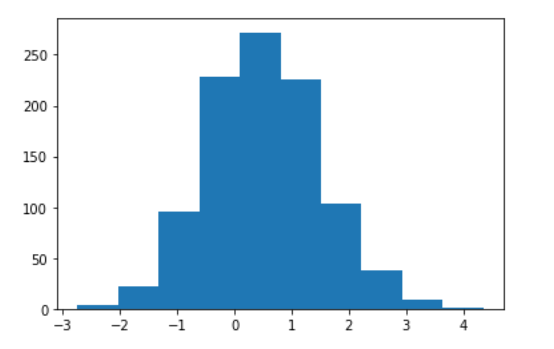1599820920

## How Bayesian Can Complement Frequentist.

For many years, academics have been using so-called frequentist statistics to evaluate whether experimental manipulations have `significant` effects.

Frequentist statistic is based on the concept of hypothesis testing, which is a mathematical based estimation of whether your results can be obtained by chance. The lower the value, the more significant it would be (in frequentist terms). By the same token, you can obtain non-significant results using the same approach. Most of these “negative” results are disregarded in research, although there is tremendous added value in also knowing what manipulations do not have an effect. But that’s for another post ;)

Thing is, in such cases where no effect can be found, frequentist statistics are limited in their explanatory power, as I will argue in this post.

Below, I will be exploring one limitation of frequentist statistics, and proposing an alternative method to frequentist hypothesis testing: `Bayesian` statistics. I will not go into a direct comparison between the two approaches. There is quite some reading out there, if you are interested. I will rather explore how why the frequentist approach presents some shortcomings, and how the two approaches can be complementary in some situations (rather than seeing them as mutually exclusive, as sometimes argued).

This is the first of two posts, where I will be focusing on the inability of frequentist statistics to disentangle between the **absence of evidence **and the evidence of absence.

## Absence of evidence vs evidence of absence

### Background

In the frequentist world, statistics typically output some statistical measures (t, F, Z values… depending on your test), and the almighty p-value. I discuss the limitations of only using p-values in another post, which you can read to get familiar with some concepts behind its computation. Briefly, the p-value, if significant (i.e., below an arbitrarily decided threshold, called alpha level, typically set at 0.05), determines that your manipulation most likely has an effect.

However, what if (and that happens a lot), your p-value is > 0.05? In the frequentist world, such p-values do not allow you to disentangle between an absence of evidence and an evidence of absence of effect.

Let that sink in for a little bit, because it is the crucial point here. In other words, frequentist statistics are pretty effective at quantifying the presence of an effect, but are quite poor at quantifying evidence for the absence of an effect. See here for literature.

The demonstration below is taken from some work that was performed at the Netherlands Institute for Neuroscience, back when I was working in neuroscience research. A very nice paper was recently published on this topic, that I encourage you to read. The code below is inspired by the paper repository, written in R.

#bayesian #data-science #python #statistics #bayesian-statistics1599632274

## Top 8 Open Source Tools For Bayesian Networks

Bayesian Network, also known as Bayes network is a probabilistic directed acyclic graphical model, which can be used for time series prediction, anomaly detection, diagnostics and more.

#bayesian #machine-learning #artificial-intelligence1597409160

## KDnuggets™ News 20:n29, Jul 29: Easy Guide To Data Preprocessing

In this issue - an easy guide to data preprocessing in Python; Monitoring Apache Spark with a better Spark UI; Computational Linear Algebra for Coders: The Free Course; Labelling Data Using Snorkel; Bayesian Statistics; and more.

#kdnuggets 2020 issues #apache spark #bayesian #data preprocessing #linear algebra #python1597169700

## Facebook Uses Bayesian Optimization to Conduct Better Experiments

Hyperparameter optimization is a key aspect of the lifecycle of machine learning applications. While methods such as grid search are incredibly effective for optimizing hyperparameters for specific isolated models, they are very difficult to scale across large permutations of models and experiments. A company like Facebook operates thousands of concurrent machine learning models that need to be constantly tuned. To achieve that, Facebook engineering teams need to regularly conduct A/B tests in order to determine the right hyperparameter configuration. Data in those tests is difficult to collect and they are typically conducted in isolation of each other which end up resulting in very computationally expensive exercises. One of the most innovative approaches in this area came from a team of AI researchers from Facebook who published a paper proposing a method based on Bayesian optimization to adaptively design rounds of A/B tests based on the results of prior tests.

#### Why Bayesian Optimization?

Bayesian optimization is a powerful method for solving black-box optimization problems that involve expensive function evaluations. Recently, Bayesian optimization has evolved as an important technique for optimizing hyperparameters in machine learning models. Conceptually, Bayesian optimization starts by evaluating a small number of randomly selected function values, and fitting a Gaussian process (GP) regression model to the results. The GP posterior provides an estimate of the function value at each point, as well as the uncertainty in that estimate. The GP works well for Bayesian optimization because it provides excellent uncertainty estimates and is analytically tractable. It provides an estimate of how an online metric varies with the parameters of interest.

Let’s imagine an environment in which we are conducting random and regular experiments on machine learning models. In that scenario, Bayesian optimization can be used to construct a statistical model of the relationship between the parameters and the online outcomes of interest and uses that model to decide which experiments to run. The concept is well illustrated in the following figure in which each data marker corresponds to the outcome of an A/B test of that parameter value. We can use the GP to decide which parameter to test next by balancing exploration (high uncertainty) with exploitation (good model estimate). This is done by computing an acquisition function that estimates the value of running an experiment with any given parameter value.The fundamental goal of Bayesian optimization when applied to hyperparameter optimization is to determine how valuable is an experiment for a specific hyperparameter configuration. Conceptually, Bayesian optimization works very efficiently for isolated models but its value proposition is challenged when used in scenarios running random experiments. The fundamental challenge is related to the noise introduced in the observations.

#bayesian #facebook #machine learning #modeling #optimization1595988780

## PMF for Recommender Systems

Automated recommender systems are commonly used to provide users with product suggestions that would be of their interest based on existing data about preferences. The literature describes different types of recommender systems. However, we will highlight two major categories and then expand further on the second one:

Content-based filtering: These make use of user preferences in order to make new predictions. When a user provides explicit information about his/her preferences, this is recorded and used by the system to automatically make suggestions. Many of the websites and social media that we commonly use everyday fall into this category.

Collaborative filtering: What happens when there isn’t enough information provided by a user to make item recommendations? Well, in these cases we can use data provided by other users with similar preferences. Methods within this category make use of the history of past choices of a group of users in order to elicit recommendations.

Whereas in the first case it is expected for a given user to build a profile that clearly states preferences, in the second scenario this information may not be fully available, but we expect our system to still be able to make recommendations based on evidence that similar users provide. A method, known as Probabilistic Matrix Factorization, or PMF for short, is commonly used for collaborative filtering, and will be the topic of our discussion for the remainder of this article. Let us now delve into the details of this algorithm as well as its intuitions.

## Probabilistic Matrix Factorization Explained

Let’s suppose we have a set of users _u_1, _u_2, _u_3 … _u_N who rate a set of items _v_1, _v_2, _v_3 … _v_M. We can then structure the ratings as a matrix R of N rows and _M _columns, where N is the number of users and M is the number of items to rate.Ratings mapping. It can be thought of as a matrix where each user (rows) rates a number of items (columns)

One important trait of the R matrix is that it is sparse. That is, only some of its cells will have a non-empty rating value, while others will not. For a given user A, the system should be able to offer item recommendations based on his/her preferences as well as the choices made by similar users. However, it is not necessary for user A to have explicitly rated a particular item for it to be recommended. Other users with similar preferences will make up for the missing data about user A. This is why _Probabilistic Matrix Factorization _falls into the category of collaborative filtering recommender systems.

Let’s think for a moment about a recommender system for movies. Imagine what things would be like if we were required to watch and rate every single movie that shows during a particular season. That would be pretty impractical, wouldn’t it? We simply lack the time to do so.

Given that not all users are able to rate all items available, we must find a way to fill in the information gaps of the R matrix and still be able to offer relevant recommendations. PMF tackles this problem by making use of ratings provided by similar users. Technically speaking, it makes use of some principles of Bayesian learning that are also applicable in other scenarios where we have scarce or incomplete data.

The R matrix can be estimated by using two low-rank matrices U and V as shown below: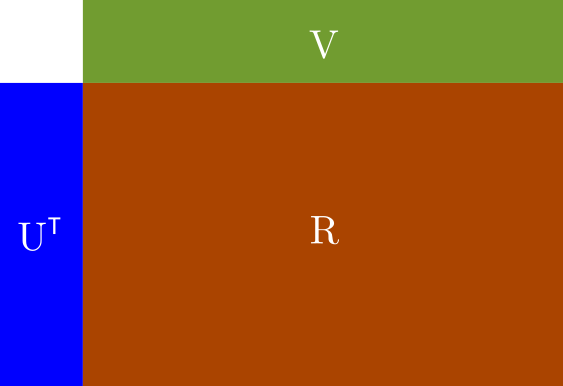Components of R matrix

Here, **U**T is an N_x_D matrix where N is the number of registered users, and D is the rank. V is a D_x_M matrix, where M is the number of items to rate. Thus the _N_x_M _ratings matrix R can be approximated by means of: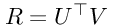Equation 1: R expression

Our job from now on is to find **U**T and V which in turn, will become the parameters of our model. Because U and V are low-rank matrices, PMF is also known as a low-rank matrix factorization problem. Furthermore, this particular trait of the U and V matrices makes PMF scalable even for datasets containing millions of records.

PMF takes its intuitions from Bayesian learning for parameter estimation. In general, we can say that in Bayesian inference, our aim is to find a posterior distribution of the model parameters by resorting to Bayes rule: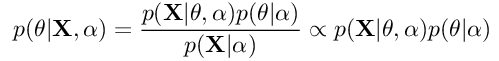Equation 2: Bayes rule for inference of parameters

Here, X is our dataset, θ is the parameter or parameter set of the distribution. α is the hyperparameter of the distribution. p(θ|X,α) is the posterior distribution, also known as a-posteriori. p(X|θ,α) is the likelihood, and p(θ|α) is the prior. The whole idea of the training process is that as we get more information about the data distribution, we will adjust the model parameter θ to fit the data. Technically speaking, the parameters of the posterior distribution will be plugged into the prior distribution for the next iteration of the training process. That is, the posterior distribution of a given training step will eventually become the prior of the next step. This procedure will be repeated until there is little variation in the posterior distribution p(θ|X,α) between steps.

Now let’s go back to our intuitions for PMF. As we stated earlier, our model parameters will be U and V_, whereas R will be our dataset. Once trained, we will end up with a revised *_R** matrix that will also contain ratings for user-item cells that were originally empty in R. We will use this revised ratings matrix to make predictions.

#bayesian #probabilistic #machine-learning #pmf #deep learning1594848120

## Quick Uncertainty Estimates for COVID19 Excess Mortality

Note from the editors:Towards Data Science_ is a Medium publication primarily based on the study of data science and machine learning. We are not health professionals or epidemiologists, and the opinions of this article should not be interpreted as professional advice. To learn more about the coronavirus pandemic, you can click here._

F

or a recent story The Economist has gathered large amounts of time series data to perform COVID “excess deaths” analysis per country.

The used method was fairly straight forward:

• Calculate weekly average deaths per country across all years pre 2020 contained in the dataset
• Compute difference between actual weekly deaths after the COVID outbreak and “predicted” average weekly deaths
• Sum up from onset of the pandemic to obtain total excess deaths.
• Compare with COVID related deaths as reported by national statistical offices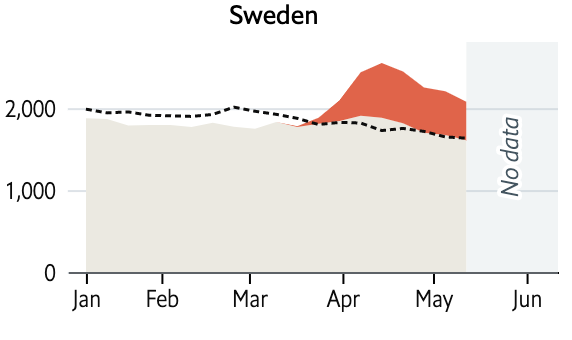Reported COVID deaths vs prediction in Sweden as per the Economist’s modelling

Although an interesting piece of data-journalism, from a Data Science point of view there are two major question-marks around their approach:

• Any method that tries to built a counterfactual (ie what if) result based on historical observations will hinge crucially on the choice of model. How do we know that the weekly average model is reasonable?
• How do we know that the observed difference is not just due to sampling error? (ie by chance). Or more specifically, how can we pin down the full posterior distribution of excess deaths more accurately?

So in in what follows I will try to quickly address these question using off-the shelf forecasting tools. Fortunately, The Economist has published all the data used for this article on github, so we can get started straight away.

# Descriptive Analysis

Quick analysis of the provided data indicates, that a lot of work has gone into building the time series, but that there are still obvious challenges around unifying data provided from national sources up to a common weekly aggregation level: Figure 1 suggests, that we have missing data, and different historical horizons across countries.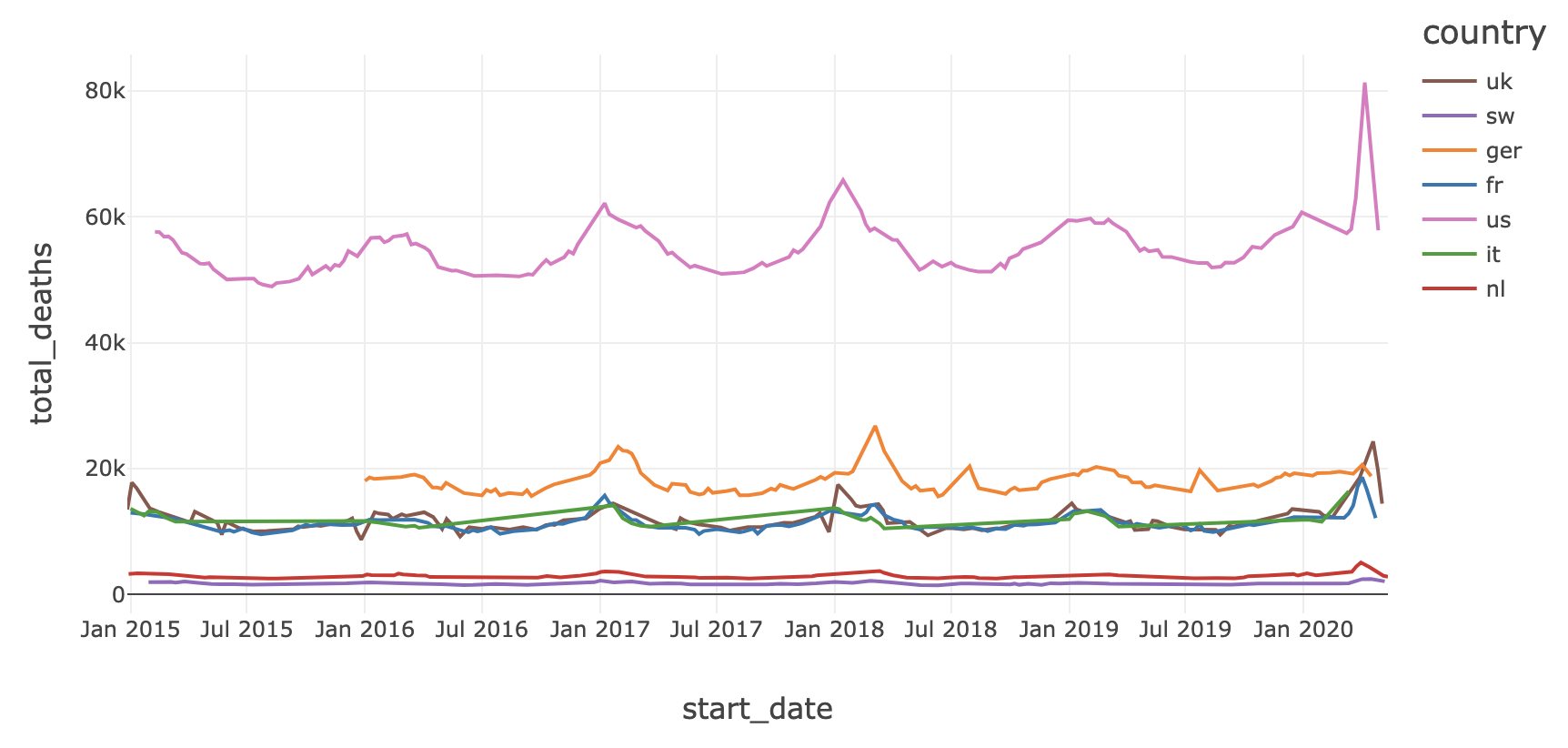Figure 1: weekly total deaths for six countries. start_date indicates first day of the respective week.

Although this might pose some modeling challenge further down the line (eg missing data, doesn’t go well with ARIMA) let us first remark on the general patterns across the processes: Firstly, we can see a pronounced yearly pattern, with annual peeks around the winter months (and around March for Germany). Secondly, we clearly see peaks in first quarter 2020 which coincide with the outbreak COVID pandemic. Thirdly, and more subtly, there are long term trends in death counts, driven by growing populations and a roughly constant mortality rate.

#covid19 #timeseries #bayesian #data-science #data analysis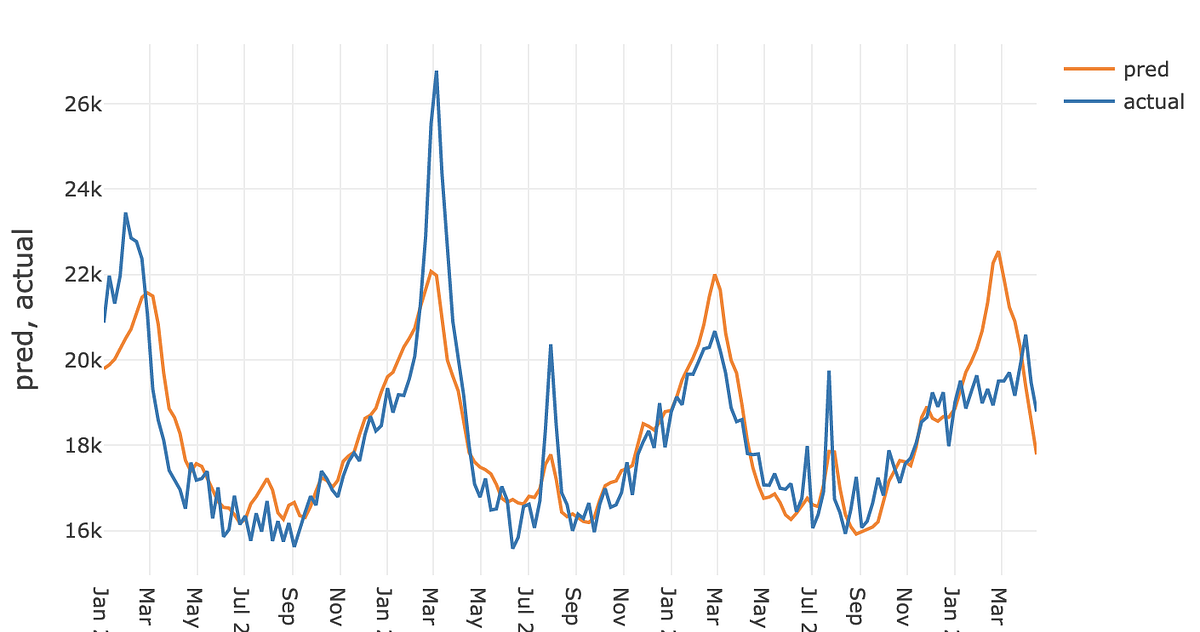1592913720

## 4 Free Math Courses to do and Level up your Data Science Skills

Just as there is no Data Science without data, there’s no science in data without mathematics. Strengthening your foundational skills in math will level you up as a data scientist that will enable you to perform with greater expertise.
When I got into Data Science and Machine Learning, anything related to math and statistics was something I had visited for the last time around 10 years ago, and I reckon that’s probably why I found it so hard at first. It took me lots of hours of reading and watching videos to get some ground understanding of how things happen for a lot of the tools we daily use in the industry. However, it got to a point where I felt the need for developing a solid understanding of what was happening underneath all those “imports” and “fits” I was doing on my Jupyter Notebook. So I decided it was time to wipe the dust off my math knowledge.

#bayesian #edx #probability #python #statistics